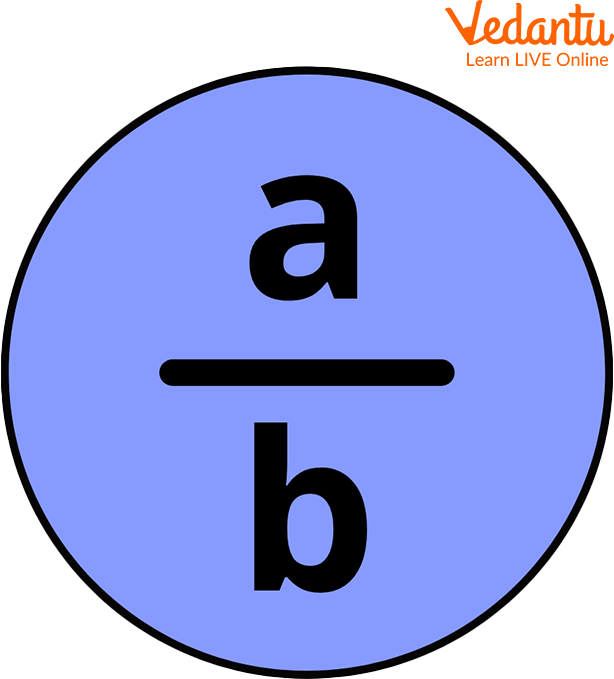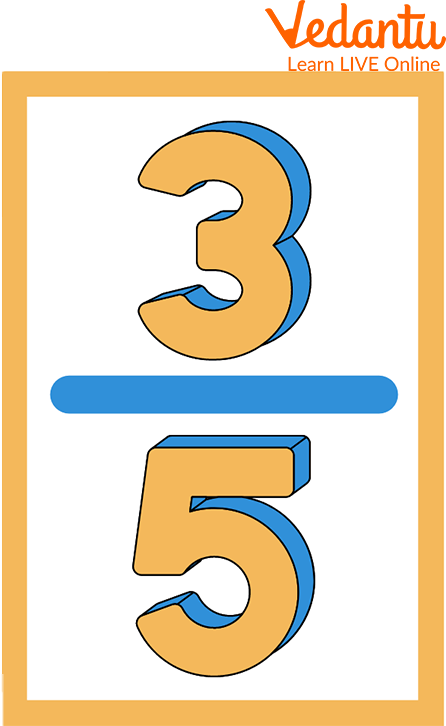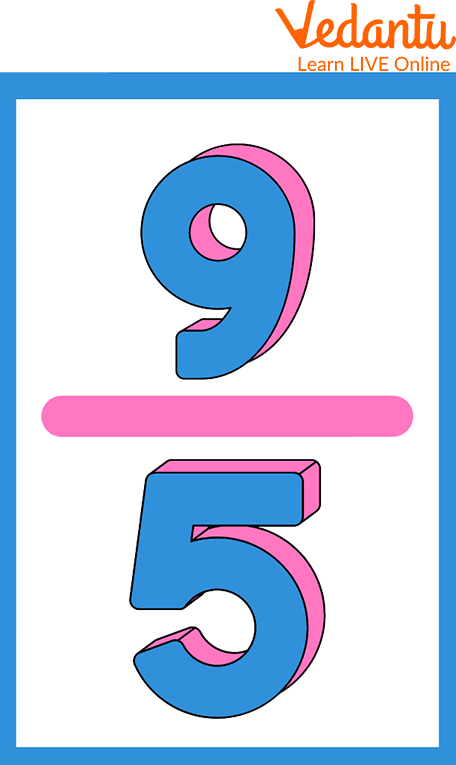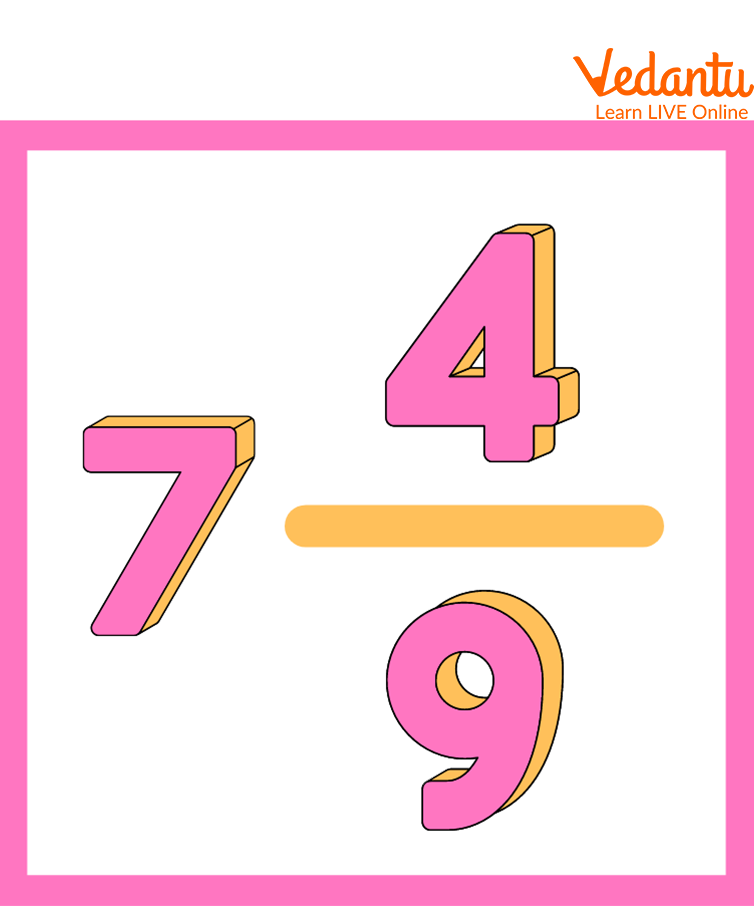Courses
Courses for Kids
Free study material
Free LIVE classes
More

# Multiplication of FractionsLIVE
Join Vedantu’s FREE Mastercalss

## Introduction to Multiplying Fractions

We know how important it is to learn about fractions because we often have their use in our daily lives. To understand fractions multiplications, knowing about their components and parts is necessary. What do a numerator and denominator denote, and what do portions mean? Multiplying fractions begins with the numerators multiplied, followed by the denominators multiplied. If necessary, the resultant fraction is simplified further and reduced to its simplest terms. So let’s head to learning in depth about the multiplication of fractions and how a number is to be multiplied by the given fraction.

## Representation of Fraction

The first and most popular way to represent a fraction is in the form of the letter a/b. Here, a and b are referred to as the numerator and denominator, respectively. There is a line dividing the numerator and denominator.Representation of a Fraction

## Types of Fraction

Proper Fraction: A fraction where the numerator is less than the denominator is known as a proper fraction.

i.e., Numerator < DenominatorProper Fraction

Improper Fraction: A fraction where the numerator is greater than the denominator, then it is known as an improper fraction.

i.e., Numerator > Denominator

For example:Improper Fraction

Mixed Fraction: A mixed fraction combines a natural number and a fraction. It is an improper fraction.

For exampleMixed Fraction

Multiplication of Fractions

In the multiplication of fractions, to multiply a fraction by one or more natural numbers or fractions, we will follow the following steps:

(i) Convert the natural numbers (if any) to improper fractions.

(ii) Convert the mixed numbers (if any) to improper fractions.

(iii) Multiply the numerator with the numerator and denominator with the denominator, cancel the common factors of the numerator and denominator and place the product of the numerator over the denominator.

(iv) Convert into a mixed fraction if needed.

Example: Multiply: $\dfrac{1}{3} \times \dfrac{3}{5}$

$\dfrac{1 \times 3}{3 \times 5}=\dfrac{3}{15}$

Now making $\dfrac{3}{15}$ into the simplest form, we will get

$=\dfrac{1}{5}$

$\therefore \dfrac{1}{3} \times \dfrac{3}{5}=\dfrac{1}{5}$

Product of two fractions = $\dfrac{\text{Product of their numerators}}{\text{Product of their denominators}}$

## Solved Examples

Q1. A number is to be multiplied by the fraction $\dfrac{4}{5}$. But Samir, by mistake, multiplied it by $\dfrac{5}{4}$ and obtained the number 81 more than the correct one. What was the original number?

Ans: Let us assume that the number is $x$.

According to the question, on taking x common, the subtracting using LCM, we get

$\Rightarrow x \times \dfrac{5}{4} -x \times \dfrac{4}{5}=81$

Now on solving the above expression, we get

$\Rightarrow x \times\dfrac{25 - 16}{20}=81$

Now, by multiplying 81 by 20 and dividing by 9, we get

$\Rightarrow x \times \dfrac{9}{20}=81$

On Cancelling the common factors, we get

$\Rightarrow x=180$

Therefore the number is 180.

Q 2. What is $\dfrac{1}{2}$ of $\dfrac{10}{7}$ in fraction?

Ans: $\dfrac{1}{2}$ of $\dfrac{10}{7}$

$=\dfrac{1}{2} \times \dfrac{10}{7}$

(Find the common factor. Here it is 2 and 10).

After cancelling the common factors, you will get

$=1 \times \dfrac{5}{7}$

Therefore, the answer is $\dfrac{5}{7}$

## Practice Problems

Q 1. Find the value of fraction A, if fraction A is thrice fraction B. The product of fractions A and B is $\dfrac{3}{49}$.

Ans: Fraction $B$ is $\dfrac{1}{7}$ and fraction $A$ is $\dfrac{3}{7}$

Q 2. What is $\dfrac{1}{2}$ of $\dfrac{3}{4}$ in fraction form?

Ans: $\dfrac{3}{8}$

Q 3. What will be the product of $\dfrac{5}{4} \times \dfrac{5}{2} \times \dfrac{5}{3}$?

Ans: $5 \dfrac{5}{24}$

## Summary

We learned and understood the concept of fractions by knowing the use of its components and the calculations done with fractions terms. We also did some questions that made our concept understanding clearer and better. The first step is to multiply the two numerators. The second step is to multiply the two denominators. Finally, simplify the new fractions. The fractions can be simplified by factoring common factors in the numerator and denominator.

Last updated date: 17th Sep 2023
Total views: 92.1k
Views today: 1.92k

## FAQs on Multiplication of Fractions

1. Who introduced the concept of fractions?

As early symbols for one part of two, one part of three, one part of four, and so on, the earliest fractions were reciprocals of integers. Egyptians divided fractions using slightly different techniques about 4000 years ago. Around 1000 BC, the Egyptians began using Egyptian fractions.

2. Where in our general life do we use fractions?

We regularly utilise fractions in our daily routines without realising them because they are such an essential aspect of existence. Everything around us uses fractions, whether you're buying half a dozen bananas or making a delicious chocolate cake using $\dfrac{3}{4}$ cup flour and $\dfrac{1}{4}$ cup milk.

3. How to teach Multiplication of Fractions?

The following methods can be used to teach fraction multiplication:

• Make students understand the use and process of fraction multiplication. To introduce the concept, use visual models as much as possible.

• After teaching a concept, use multiplying fractions worksheets.